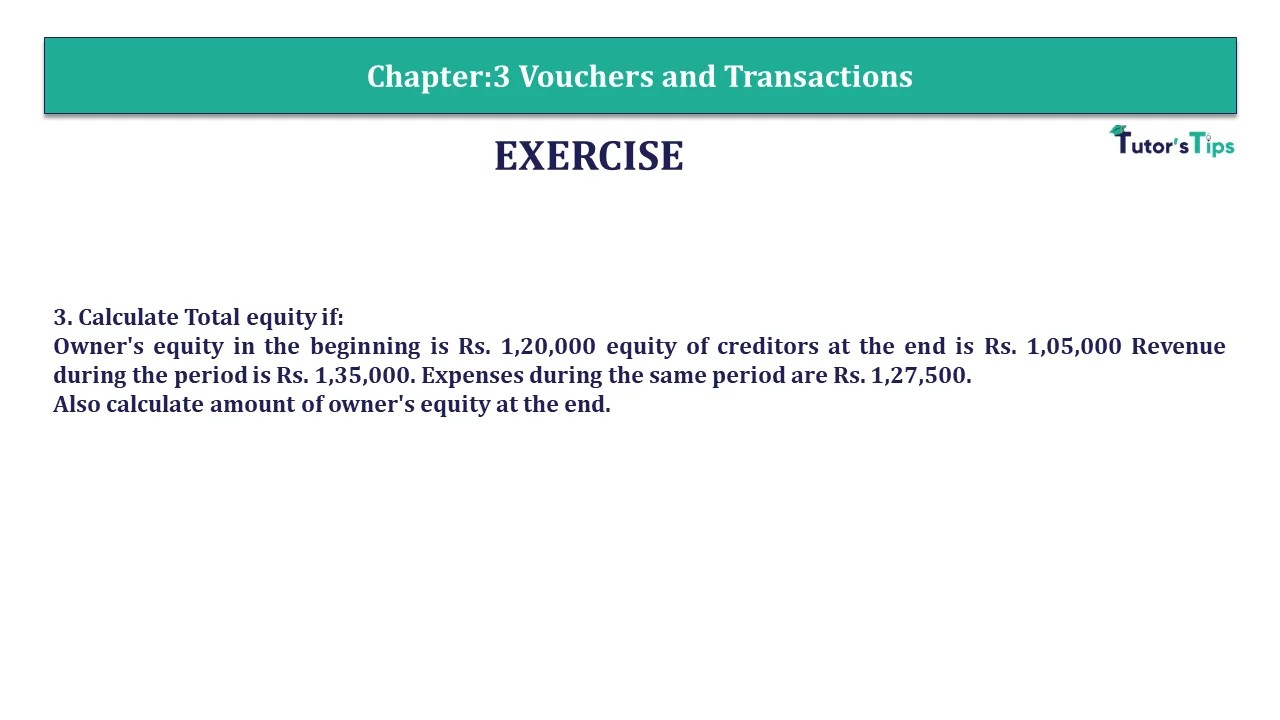# Question 3 Chapter 3 – Unimax Publications of Class 11Q-3- CH-3 Solution

Question 3 Chapter 3 – Unimax

3. Calculate Total equity if:
Owner’s equity in the beginning is Rs. 1,20,000 equity of creditors at the end is Rs. 1,05,000 Revenue during the period is Rs. 1,35,000. Expenses during the same period are Rs. 1,27,500.
Also calculate amount of owner’s equity at the end.

## The solution of Question 3 Chapter 3 – Unimax:

 Total Equity =Owner’s Equity + Creditor’s equity = (Opening Owner’s equity + Revenue – Expenses) + Creditors equity =(1,20,000+ 1,35,000-1,27,500)+1,05,000 = 1,27,500+1,05,000 = 2,32,500

This is all about the Question 3 Chapter 3 – Unimax. You can check out the following article to better understand:

What is Accounting Equation | Example

You Can also read all above articles in Hindi on our Hindi Website

What is the Accounting Equation- In Hindi

Comment if you have any doubt in the Question 3 Chapter 3 – Unimax.

You can also Check out the solved question of other Chapters: –

## Advanced Accountancy – Unimax Class 11 – 2021 – Solution.

Part-I

• Chapter No. 1 – Introduction of Accounting
• Chapter No. 2 – Theory Base of Accounting
• Chapter No. 3 – Vouchers and Transactions
• Chapter No. 4 – Journal
• Chapter No. 5 – Goods and Services Tax (GST) : An Introduction
• Chapter No. 6 – Ledger
• Chapter No. 7 – Special Purpose Book – Cash Book
• Chapter No. 8 – Other Subsidiary Books
• Chapter No. 9 – Trial Balance
• Chapter No. 10 – Rectification of Errors
• Chapter No. 11 – Depreciation
• Chapter No. 12 – Provision and Reserves
• Chapter No. 13 – Bank Reconlciliation Statement
• Chapter No. 14 – Bills of Exchange

Students may Choose only one part from the Part II and Part III

Part-II

• Chapter No. 15 – Financial Statements (Without Adjustments)
• Chapter No. 16 – Financial Statements (With Adjustments)
• Chapter No. 17 – Accounts from Incomplete Records – Single Entry System

Part-III

• Chapter No. 18 – Introduction to Compurters and Accounting information System
• Chapter No. 19 – Computerised Accounting
• Chapter No. 20 – Accounting Software : Tally
• Chapter No. 21 – Data Base System
• Chapter No. 22 – Concept of Entity and Relationship

You can also Check out the other Books’ Solution: –

error: Content is protected !!